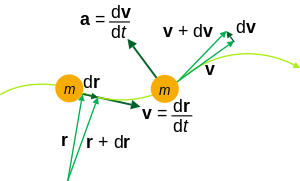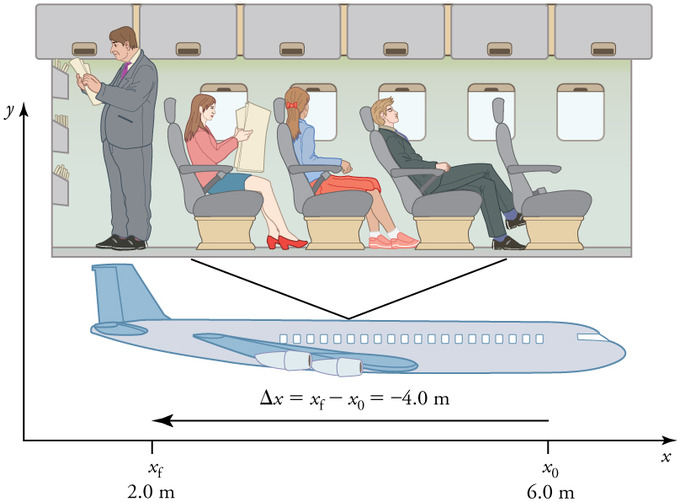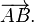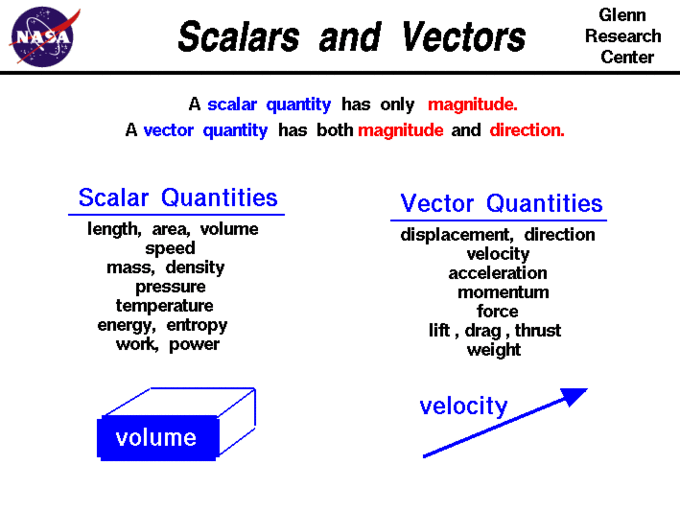# Defining Kinematics

Kinematics is the study of the motion of points, objects, and groups of objects without considering the causes of its motion.

### Learning Objectives

Define kinematics

### Key Takeaways

#### Key Points

• To describe motion, kinematics studies the trajectories of points, lines and other geometric objects.
• The study of kinematics can be abstracted into purely mathematical expressions.
• Kinematic equations can be used to calculate various aspects of motion such as velocity, acceleration, displacement, and time.

#### Key Terms

• kinematics: The branch of mechanics concerned with objects in motion, but not with the forces involved.

Kinematics is the branch of classical mechanics that describes the motion of points, objects and systems of groups of objects, without reference to the causes of motion (i.e., forces ). The study of kinematics is often referred to as the “geometry of motion.”

Objects are in motion all around us. Everything from a tennis match to a space-probe flyby of the planet Neptune involves motion. When you are resting, your heart moves blood through your veins. Even in inanimate objects there is continuous motion in the vibrations of atoms and molecules. Interesting questions about motion can arise: how long will it take for a space probe to travel to Mars? Where will a football land if thrown at a certain angle? An understanding of motion, however, is also key to understanding other concepts in physics. An understanding of acceleration, for example, is crucial to the study of force.

To describe motion, kinematics studies the trajectories of points, lines and other geometric objects, as well as their differential properties (such as velocity and acceleration). Kinematics is used in astrophysics to describe the motion of celestial bodies and systems; and in mechanical engineering, robotics and biomechanics to describe the motion of systems composed of joined parts (such as an engine, a robotic arm, or the skeleton of the human body).

A formal study of physics begins with kinematics. The word “kinematics” comes from a Greek word “kinesis” meaning motion, and is related to other English words such as “cinema” (movies) and “kinesiology” (the study of human motion). Kinematic analysis is the process of measuring the kinematic quantities used to describe motion. The study of kinematics can be abstracted into purely mathematical expressions, which can be used to calculate various aspects of motion such as velocity, acceleration, displacement, time, and trajectory.Kinematics of a particle trajectory: Kinematic equations can be used to calculate the trajectory of particles or objects. The physical quantities relevant to the motion of a particle include: mass m, position r, velocity v, acceleration a.

## Reference Frames and Displacement

In order to describe an object’s motion, you need to specify its position relative to a convenient reference frame.

### Learning Objectives

Evaluate displacement within a frame of reference.

### Key Takeaways

#### Key Points

• Choosing a frame of reference requires deciding where the object’s initial position is and which direction will be considered positive.
• Valid frames of reference can differ from each other by moving relative to one another.
• Frames of reference are particularly important when describing an object’s displacement.
• Displacement is the change in position of an object relative to its reference frame.

#### Key Terms

• displacement: A vector quantity that denotes distance with a directional component.
• frame of reference: A coordinate system or set of axes within which to measure the position, orientation, and other properties of objects in it.

In order to describe the motion of an object, you must first describe its position — where it is at any particular time. More precisely, you need to specify its position relative to a convenient reference frame. Earth is often used as a reference frame, and we often describe the position of objects related to its position to or from Earth. Mathematically, the position of an object is generally represented by the variable x.

### Frames of Reference

There are two choices you have to make in order to define a position variable x. You have to decide where to put x = 0 and which direction will be positive. This is referred to as choosing a coordinate system, or choosing a frame of reference. As long as you are consistent, any frame is equally valid. But you don’t want to change coordinate systems in the middle of a calculation. Imagine sitting in a train in a station when suddenly you notice that the station is moving backward. Most people would say that they just failed to notice that the train was moving — it only seemed like the station was moving. But this shows that there is a third arbitrary choice that goes into choosing a coordinate system: valid frames of reference can differ from each other by moving relative to one another. It might seem strange to use a coordinate system moving relative to the earth — but, for instance, the frame of reference moving along with a train might be far more convenient for describing things happening inside the train. Frames of reference are particularly important when describing an object’s displacement.

FRAMES OF REFERENCE by Professor Hume and Professor Donald Ivey of the University of Toronto

In this classic film, Professors Hume and Ivey cleverly illustrate reference frames and distinguish between fixed and moving frames of reference.

Frames of Reference (1960) Educational Film: Frames of Reference is a 1960 educational film by Physical Sciences Study Committee. The film was made to be shown in high school physics courses. In the film University of Toronto physics professors Patterson Hume and Donald Ivey explain the distinction between inertial and nonintertial frames of reference, while demonstrating these concepts through humorous camera tricks. For example, the film opens with Dr. Hume, who appears to be upside down, accusing Dr. Ivey of being upside down. Only when the pair flip a coin does it become obvious that Dr. Ivey — and the camera — are indeed inverted. The film’s humor serves both to hold students’ interest and to demonstrate the concepts being discussed. This PSSC film utilizes a fascinating set consisting of a rotating table and furniture occupying surprisingly unpredictable spots within the viewing area. The fine cinematography by Abraham Morochnik, and funny narration by University of Toronto professors Donald Ivey and Patterson Hume is a wonderful example of the fun a creative team of filmmakers can have with a subject that other, less imaginative types might find pedestrian. Producer: Richard Leacock Production Company: Educational Development Corp. Sponsor: Eric Prestamon

### Displacement

Displacement is the change in position of an object relative to its reference frame. For example, if a car moves from a house to a grocery store, its displacement is the relative distance of the grocery store to the reference frame, or the house. The word “displacement” implies that an object has moved or has been displaced. Displacement is the change in position of an object and can be represented mathematically as follows:

$\Delta \text{x} = \text{x}_\text{f} - \text{x}_0$

where Δx is displacement, xf is the final position, and x0 is the initial position.

shows the importance of using a frame of reference when describing the displacement of a passenger on an airplane.Displacement in Terms of Frame of Reference: A passenger moves from his seat to the back of the plane. His location relative to the airplane is given by x. The -4.0m displacement of the passenger relative to the plane is represented by an arrow toward the rear of the plane. Notice that the arrow representing his displacement is twice as long as the arrow representing the displacement of the professor (he moves twice as far).

## Introduction to Scalars and Vectors

A vector is any quantity that has both magnitude and direction, whereas a scalar has only magnitude.

### Learning Objectives

Distinguish the difference between scalars and vectors

### Key Takeaways

#### Key Points

• A vector is any quantity that has magnitude and direction.
• A scalar is any quantity that has magnitude but no direction.
• Displacement and velocity are vectors, whereas distance and speed are scalars.

#### Key Terms

• scalar: A quantity that has magnitude but not direction; compare vector.
• vector: A directed quantity, one with both magnitude and direction; the between two points.

What is the difference between distance and displacement? Whereas displacement is defined by both direction and magnitude, distance is defined by magnitude alone. Displacement is an example of a vector quantity. Distance is an example of a scalar quantity. A vector is any quantity with both magnitude and direction. Other examples of vectors include a velocity of 90 km/h east and a force of 500 newtons straight down.

Scalars and Vectors: Mr. Andersen explains the differences between scalar and vectors quantities. He also uses a demonstration to show the importance of vectors and vector addition.

In mathematics, physics, and engineering, a vector is a geometric object that has a magnitude (or length) and direction and can be added to other vectors according to vector algebra. The direction of a vector in one-dimensional motion is given simply by a plus (+) or minus (−) sign. A vector is frequently represented by a line segment with a definite direction, or graphically as an arrow, connecting an initial point A with a terminal point B, as shown in.Vector representation: A vector is frequently represented by a line segment with a definite direction, or graphically as an arrow, connecting an initial point A with a terminal point B.

Some physical quantities, like distance, either have no direction or no specified direction. In physics, a scalar is a simple physical quantity that is not changed by coordinate system rotations or translations. It is any quantity that can be expressed by a single number and has a magnitude, but no direction. For example, a 20ºC temperature, the 250 kilocalories (250 Calories) of energy in a candy bar, a 90 km/h speed limit, a person’s 1.8 m height, and a distance of 2.0 m are all scalars, or quantities with no specified direction. Note, however, that a scalar can be negative, such as a −20ºC temperature. In this case, the minus sign indicates a point on a scale rather than a direction. Scalars are never represented by arrows. (A comparison of scalars vs. vectors is shown in. )Scalars vs. Vectors: A brief list of quantities that are either scalars or vectors.